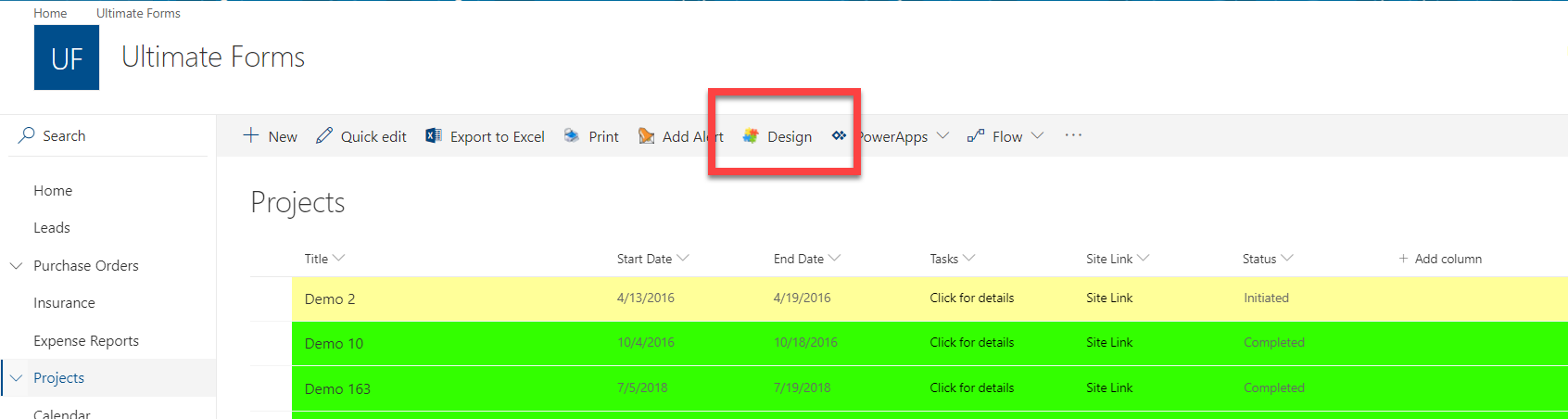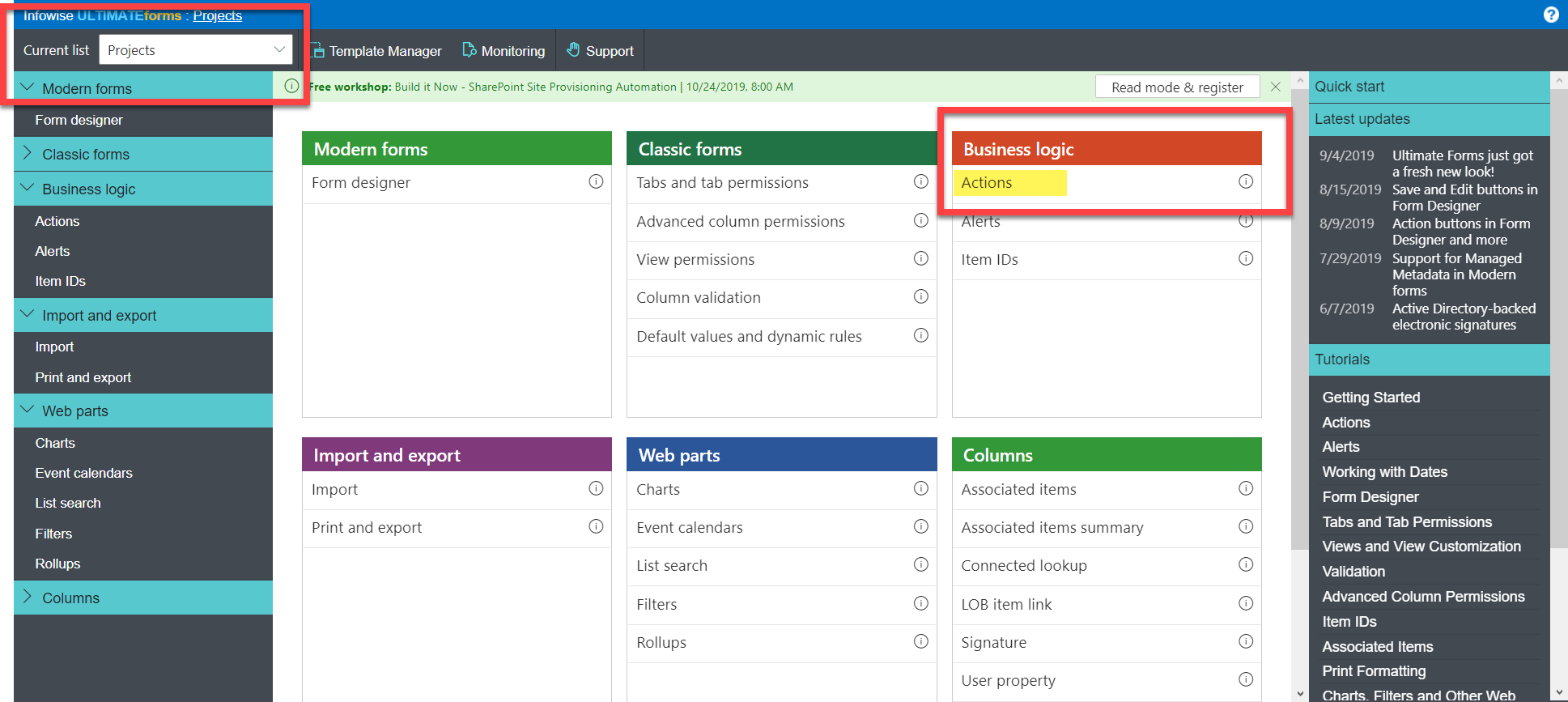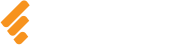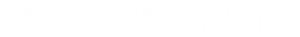# Tutorial

## AND / OR Conditions

Multiple AND / OR conditions can be chained together. It is critical to understand how the system interprets these rules.

Build a test example like the one demonstrated to experiment with chained conditions or study the pattern described below.

In some cases it is necessary to use more than one action to handle all possible conditions.

Note: This video shows creating Actions with an older version of Ultimate Forms. The process remains the same, but the way you access Ultimate Forms has changed a little.

If you’re in the SharePoint Modern experience, the link for Ultimate Forms will be on the menu bar of your list or library (if it’s not visible, you can also get to Ultimate Forms via the site contents).Verify you’re working in the list or library you want.

To open the Actions feature, click the Actions link in the Business Logic section of the menu. Once you’re in the tool, the process is the same as shown in the video.

## Be careful when chaining multiple AND and OR conditions!

When chaining together multiple conditions, each new condition is handled in comparison to the entire preceding set of condtions.

Imagine that the whole statement preceding the new condition always gets enclosed in parenthesis like this:

(((((CONDITION 1) OR CONDITION 2) AND CONDITION 3) OR CONDITION 4) OR CONDITION 5)

If you chain only AND or OR conditions together things work clearly, but you can get tripped when combining AND and OR conditions.

If you need to evaluate complex condition using both AND and OR operators here is some strategy to consider:

• Break one action up into multiple actions. Handle your OR situations by creating an action for each situation that you cover normally by using the OR operator.
• Putting the OR conditions first sometimes can make it easier to build your logic.
• Create a calculated field to evaluate the composite conditions and return YES or NO. You can fully control the nesting of conditions with this approach.

EXAMPLES:

Example A:

AND Var1 = 1

AND Var2 = 2

• Evaluates as ((Var1 = 1) AND Var2 = 2)

Example B:

AND Var1 = 1

OR Var2 = 2

• Evaluates as ((Var1 = 1) OR Var2 = 2)

Example C:

AND Var1 = 1

AND Var2 = 2

OR Var3 = 3

• Evaluates as (((Var1 = 1) AND Var2 = 2) OR Var3 = 3)

Example D:

AND Var1 = 1

OR Var2 = 2

AND Var3 = 3

OR Var4 = 4

• Evaluates as ((((Var1 = 1) OR Var2 = 2) AND Var3 = 3) OR Var4 = 4)

Example E:

AND Var1 = 1

AND Var2 = 2

OR Var3 = 3

AND Var4 = 4

OR Var5 = 5

• Evaluates as (((((Var1 = 1) AND Var2 = 2) OR Var3 = 3) AND Var4 = 4) OR Var5 = 5)

Example F:

CREATE this logic:

Evaluate (Var1 = 1) AND (Var2 = 2) AND (Var3 = 3 OR Var4 = 4)

In this case, put the OR conditions first.

AND Var3 = 3

OR Var4 = 4

AND Var1 = 1

AND Var2 = 2

Another approach:

Make TWO actions to easily cover the OR situation:

Action 1:

AND Var1 = 1

AND Var2 = 2

AND Var3 = 3

Action 2:

AND Var1 = 1

AND Var2 = 2

AND Var4 = 4

Example G:

CREATE this logic:

Evaluate (Var1 = 1 OR Var2 = 2) AND (Var3 = 3 OR Var4 = 4)

Split this into multiple actions:

Action 1

AND Var1 = 1

AND Var3 = 3

Action 2

AND Var1 = 1

AND Var4 = 4

Action 3

AND Var2 = 2

AND Var3 = 3

Action 4

AND Var2 = 2

AND Var4 = 4

## Summary

Using conditions in actions is an critical part of the Infowise workflow functionality. Be certain to have a firm grasp on how chained conditions are interpreted to avoid unexpected headaches!